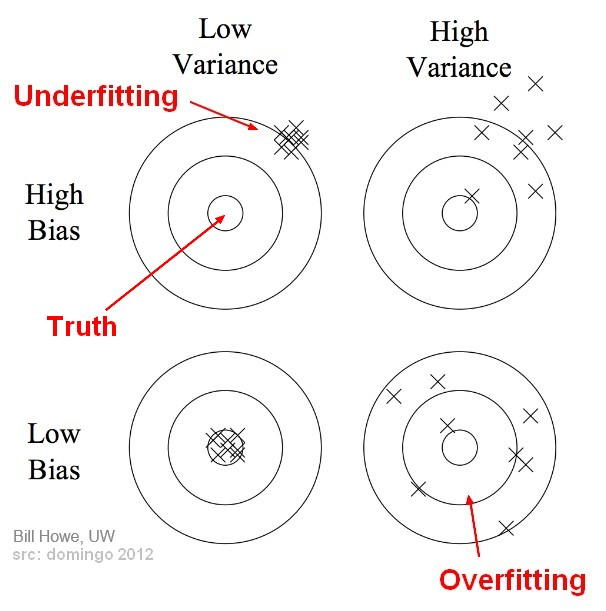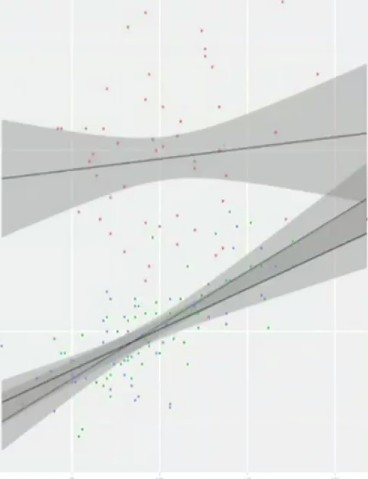# Statistics - Raw score

A (raw|observed) score is the score

• in the original scale.
• not predicted (calculated)
• just observed

## Formula

(Classical test|True score) theory asserts that raw scores are influenced by:

$$\href{Raw Score}{Raw\,Score} = \href{True score}{True\,Score} + \href{bias}{Bias}+Error$$

where:

• True Score is the score in a perfect world
• Bias is a systematic error. Example: An instrument that will always measure with the same difference. It has an effect on the entire distribution (It shift it right or left)
• Error: is also known as Chance error, or random chance error (For example, measurement of body temperature, if someone drink a coffer before measuring its temperature)

Recommended PagesStatistics - (Variance|Dispersion|Mean Square) (MS)

The variance shows how widespread the individuals are from the average. The variance is how much that the estimate varies around its average. It's a measure of consistency. A very large variance means...Statistics - (dependent|paired sample) t-test

A dependent t-test is appropriate when: we have the same people measured twice. the same subject are been compared (ex: Pre/Post Design) or two samples are matched at the level of individual subjects...Statistics - Moderator Variable (Z) - Moderation

A moderation analysis is a multiple regression analysis. The main reason to run a moderation analysis is to demonstrate how a third variable (Z) changes the correlation between two variables (X and Y)....Statistics - Raw score

A (raw|observed) score is the score in the original scale. not predicted (calculated) just observed (Classical test|True score) theory asserts that raw scores are influenced by: bias, ...Statistics - Reliability (Reliable Measures)

How do we know how reliable our measure are. Raw scores (X) are not perfect. (Classical test|True score) theory asserts that raw scores are influenced by: bias, chance error, and true scores...Statistics - True score (Classical test theory)

The true score is the score the truth that we try to estimate. (Classical test|True score) theory asserts that raw scores are influenced by: bias, chance error, and true scores where:...Statistics - Z Score (Zero Mean) or Standard Score

Any raw score from any scale can be converted to Z scores (Z scale) In statistics, the standard score is the signed number of standard deviations by which an observation or data is above the mean. ...